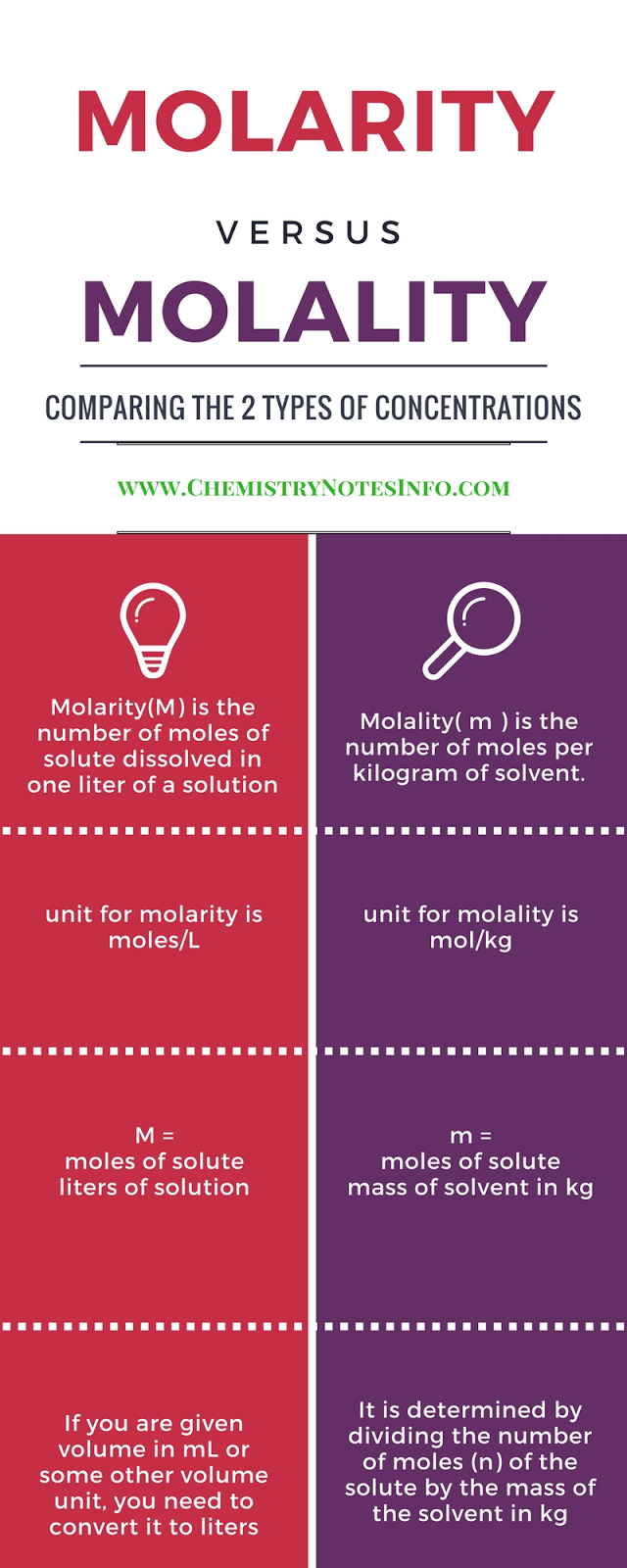# Wet-Chemistry

## What is Wet Chemistry ?

Wet Chemistry  is a branch of chemistry which deals with the study of and testing of chemicals in liquid state. Wetchemistry is also known as Bench Chemistry as many wet chemical methods are performed on chemistry laboratory benches.

# What is Normality ?

Normality is the ratio of gram equivalent weight to volume of solution in liter
where gram equivalent is the measure of reactive capacity of molecule consider for normality.

## Normality (N) :-

Gram equivalent weight  / Volume of the solution in liter.

Unit =>   N = "eq/L" (equivalent per liter)

## Aufbau Principle

According to this principle in the ground state of the atoms the orbital’s are filled in order of their increasing energies means electrons enter higher energy orbital’s  so order in which orbital’s are filled is 1s, 2s, 2p, 3s, 3p, 4s, 3d, 4p, 5s, 4d, 5p, 6s, 4f, 5d, 6p, 7s, 5f, 6d, 7p.

## Pauli Exclusion Principle

·         given by W. Pauli (1926).
·        Two electrons in an atoms can’t have same set of 4-quantum no.
·        Only two electrons may exist in same orbital and these electrons must have opposite spin.

## Hund’s Rule of Maximum Multiplicity

·        According to this rule pairing of  electron in the orbital’s belonging to the same sub-shell (p, d or f)
·        Does not take place until each orbital belonging to that sub-shell has got one electron each i.e. it is singly occupied.

# What is Molality?

It is the ratio of moles of substance to kilogram of solvent.
Where mole is weight in gram divided by molecular weight.
Molality is chemistry terminology.

## Molality (m) :-

No. of moles of the solute  Mass of solvent in Kilogram (Kg).

Unit =>   m = Moles/KgWhat is Molality

# Analytical Chemistry

Definition of Analytical Chemistry- Analytical Chemistry is the branch of chemistry deals with the study of material examination to separate out them into different components and identifying all components and how much these components present in material. There are different analytical methods and techniques to perform these tasks.
Analytical chemistry is all about, what an analytical chemist do in an analytical chemistry lab. And simply we can say that analytical chemistry is the branch of chemistry for qualitative analysis and quantitative analysis of compounds and mixtures by using different test methods and techniques like flame tests, chemical tests, precipitation, titration, chromatography, spectroscopy, separation, microscopy etc.

## Types of Analytical Methods

Analytical chemistry uses two types of methods for analysis that are classical and modern methods. Classical methods are wet chemical methods while modern methods are instrumental methods. Classical analytical methods are further subdivided into two categories i.e. 1. Classical Qualitative Analytical Methods and 2. Classical Quantitative Analytical Methods. Similarly, Modern analytical methods are further subdivided into two categories i.e. 1. Modern Qualitative Analytical Methods and 2. Modern Quantitative Analytical Methods.

## A.  Classical Analytical Methods

### 1.   Classical Qualitative Analytical Methods

Classical qualitative analytical methods uses precipitation, extraction, and distillation for separation purpose and differences in color, odor, melting point, boiling point, reactivity for identification purpose.
Some of the classical qualitative analytical methods are given below-

### 2.   Classical Quantitative Analytical Methods

Classical quantitative analytical methods uses change in mass and volume for quantification of amount of particular analyte in analytical sample.
Some of the classical quantitative analytical methods are given below-

## B.  Modern Analytical Methods

### 1.   Modern Qualitative Analytical Methods

Modern qualitative analytical methods uses chromatography, electrophoresis for separation purpose and uses instruments for identification purposes.

### 2.   Modern Quantitative Analytical Methods

Modern quantitative analytical methods uses different instruments for quantitative analysis. These instruments are based on the principles of light or heat interaction, electric or magnetic fields. Generally a single modern instrument is enough for separation, identification and quantification of an analyte.

Modern analytical methods uses different instruments so we can say that modern analytical methods are Instrumental methods. And some of them are given below-

## What is an Analytical Chemist ?

Analytical chemist is a chemical scientist who have all knowledge about analytical chemistry with other branches of chemistry like physical, organic and inorganic chemistry. They works in chemical labs or analytical chemistry laboratories to analyses different chemical compounds and mixtures.

### What does an analytical chemist do ?

Analytical Chemist analyses samples to find out different analytes. They do different experiments in chemical labs to know about exact chemical component present in sample. Analytical chemist get all information from a sample means what that sample contains and how much contains. Nowadays analytical chemist do different researches to make analysis quick and more accurate by the use of modern techniques and modern equipment and advanced software’s.

### Where does an analytical chemist work ?

Analytical chemistry work in chemical labs and analytical chemistry labs, means they conduct their experiment inside labs and then come back to analytical labs offices to interpret with experimental data.

Do you know ?

### What is an analytical balance?

Analytical balance is a lab-instrument used to determine mass of any matter very precisely. These analytical balances are very sensitive and expensive lab-instruments, and also upon the accuracy and precision of analytical balance the accuracy of lab-analysis result depends so handle these balances very carefully. Generally used analytical balances are balances with the capacity of 100 gram to 200 gram and the sensitivity of 0.1 miligram to 0.001 miligram. For any quantitative chemical analysis there is need of analytical balance for the weighing of sample for analysis and weighing of reagents for solution preparation. Analytcal balance pan used to put sample on it is enclosed in a glass-fitted case, means analytical balance sensitivity requires that it to be protected by the draft shield or enclosure...read more.

## NOTE:

If error occur=> Change website url from www.chemistrynotesinfo.blogspot.in to www.chemistrynotesinfo.com